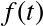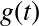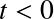$$\newcommand{\id}{\mathrm{id}}$$ $$\newcommand{\Span}{\mathrm{span}}$$ $$\newcommand{\kernel}{\mathrm{null}\,}$$ $$\newcommand{\range}{\mathrm{range}\,}$$ $$\newcommand{\RealPart}{\mathrm{Re}}$$ $$\newcommand{\ImaginaryPart}{\mathrm{Im}}$$ $$\newcommand{\Argument}{\mathrm{Arg}}$$ $$\newcommand{\norm}{\| #1 \|}$$ $$\newcommand{\inner}{\langle #1, #2 \rangle}$$ $$\newcommand{\Span}{\mathrm{span}}$$

# 6.3: Convolution

•• Contributed by Jiří Lebl
• Assistant Professor (Mathematics) at Oklahoma State University
$$\newcommand{\vecs}{\overset { \rightharpoonup} {\mathbf{#1}} }$$ $$\newcommand{\vecd}{\overset{-\!-\!\rightharpoonup}{\vphantom{a}\smash {#1}}}$$$$\newcommand{\id}{\mathrm{id}}$$ $$\newcommand{\Span}{\mathrm{span}}$$ $$\newcommand{\kernel}{\mathrm{null}\,}$$ $$\newcommand{\range}{\mathrm{range}\,}$$ $$\newcommand{\RealPart}{\mathrm{Re}}$$ $$\newcommand{\ImaginaryPart}{\mathrm{Im}}$$ $$\newcommand{\Argument}{\mathrm{Arg}}$$ $$\newcommand{\norm}{\| #1 \|}$$ $$\newcommand{\inner}{\langle #1, #2 \rangle}$$ $$\newcommand{\Span}{\mathrm{span}}$$ $$\newcommand{\id}{\mathrm{id}}$$ $$\newcommand{\Span}{\mathrm{span}}$$ $$\newcommand{\kernel}{\mathrm{null}\,}$$ $$\newcommand{\range}{\mathrm{range}\,}$$ $$\newcommand{\RealPart}{\mathrm{Re}}$$ $$\newcommand{\ImaginaryPart}{\mathrm{Im}}$$ $$\newcommand{\Argument}{\mathrm{Arg}}$$ $$\newcommand{\norm}{\| #1 \|}$$ $$\newcommand{\inner}{\langle #1, #2 \rangle}$$ $$\newcommand{\Span}{\mathrm{span}}$$

We said that the Laplace transformation of a product is not the product of the transforms. All hope is not lost however. We simply have to use a different type of a “product.” Take two functions $$f(t)$$ and $$g(t)$$ defined for $$t \geq 0$$, and define the convolution3 of $$f(t)$$ and $$g(t)$$ as

$(f \ast g)(t) \overset{\rm{def}}{=} \int_0^t f(\tau)g(t- \tau)d\tau .$

As you can see, the convolution of two functions of $$t$$ is another function of $$t$$.

Example $$\PageIndex{1}$$

Take $$f(t)=e^t$$ and $$g(t) = t$$ for $$t \geq 0$$. Then

$(f \ast g)(t) = \int_0^t e^{\tau}(t- \tau)d\tau = e^t-t-1.$

To solve the integral we did one integration by parts.

Example $$\PageIndex{2}$$

Take $$f(t)=\sin(\omega t)$$ and $$g(t)=\cos(\omega t)$$ for $$t \geq 0$$. Then

$(f \ast g)(t) = \int_0^t \sin(\omega \tau)\cos(\omega (t- \tau))d\tau.$

We apply the identity

$\cos(\theta)\sin(\psi)= \dfrac{1}{2}(\sin(\theta + \psi)-\sin(\theta -\psi ))$

Hence,

\begin{align} (f \ast g)(t) & = \int_0^t \dfrac{1}{2}(\sin(\omega t) - \sin(\omega t-2 \omega \tau )) d\tau \\ &=\left[ \dfrac{1}{2} \tau \sin(\omega t)+\dfrac{1}{4\omega} \cos(2\omega \tau -\omega t) \right]_{\tau =0}^t \\ &= \dfrac{1}{2} t \sin(\omega t). \end{align}

The formula holds only for $$t \geq 0$$. We assumed that $$f$$ and $$g$$ are zero (or simply not deﬁned) for negative $$t$$.

The convolution has many properties that make it behave like a product. Let $$c$$ be a constant and $$f$$, $$g$$, and $$h$$ be functions then

\begin{align} f*g &= g* f \\ (c\,f)*g &= f*(c\,g)=c\,(f*g) \\ (f*g)*h&=f*(g*h) \end{align}

The most interesting property for us, and the main result of this section is the following theorem.

Theorem 6.3.1:

Let $$f(t)$$ and $$g(t)$$ be of exponential type, then

$\mathcal\{(f \ast g)(t)\}= \mathcal{L} \left\{ \int_0^t f(\tau)g(t- \tau)d\tau \right\}=\mathcal{L}\{f(t)\} \mathcal{L} \{g(t)\}.$

In other words, the Laplace transform of a convolution is the product of the Laplace transforms. The simplest way to use this result is in reverse.

Example $$\PageIndex{3}$$

Suppose we have the function of $$s$$ deﬁned by

$\dfrac{1}{(s+1)s^2}=\dfrac{1}{s+1}\dfrac{1}{s^2}.$

We recognize the two entries of Table 6.2. That is

$\mathcal{L}^{-1} \left\{ \dfrac{1}{s+1} \right\}=e^{-t}~~~~~~ {\rm{and}}~~~~~~ \mathcal{L}^{-1} \left\{ \dfrac{1}{s^2} \right\}=t.$

Therefore,

$\mathcal{L}^{-1} \left\{ \dfrac{1}{s+1} \dfrac{1}{s^2} \right\}=\int_0^t \tau e^{-(t- \tau)}d\tau =e^{-t}+t-1.$

The calculation of the integral involved an integration by parts.

## Solving ODEs

The next example demonstrates the full power of the convolution and the Laplace transform. We can give the solution to the forced oscillation problem for any forcing function as a definite integral.

Example $$\PageIndex{4}$$

Find the solution to

$x''+\omega_0^2x=f(t),~~~~~x(0)=0,~~~~~x'(0)=0,$

for an arbitrary function $$f(t)$$.

We ﬁrst apply the Laplace transform to the equation. Denote the transform of $$x(t)$$ by $$X(s)$$ and the transform of $$f(t)$$ by $$F(s)$$ as usual.

$s^2X(s)+ \omega_0^2X(s)=F(s),$

or in other words

$X(s)=F(s) \dfrac{1}{s^2+ \omega_0^2}.$

We know

$\mathcal{L}^{-1} \left\{ \dfrac{1}{s^2+ \omega_0^2} \right\}= \dfrac{\sin(\omega_0 t)}{\omega_0}.$

Therefore,

$x(t)= \int_0^tf(\tau)\dfrac{\sin(\omega_0( t-\tau))}{\omega_0}d\tau ,$

or if we reverse the order

$x(t)= \int_0^t \dfrac{\sin(\omega_0 \tau)}{\omega_0}f(t-\tau)d\tau .$

Let us notice one more feature of this example. We can now see how Laplace transform handles resonance. Suppose that $$f(t)=\cos(\omega_0t)$$. Then

$x(t)= \int_0^t \dfrac{\sin(\omega_0 \tau)}{\omega_0}\cos(\omega_0(t-\tau))d\tau = \dfrac{1}{\omega_0}\int_0^t \sin(\omega_0 \tau)\cos(\omega_0(t-\tau))d\tau .$

We have computed the convolution of sine and cosine in Example 6.3.2. Hence

$x(t)=\left( \dfrac{1}{\omega_0}\right) \left( \dfrac{1}{2}t\sin(\omega_0t)\right) = \dfrac{1}{2\omega_0} \sin(\omega_0t).$

Note the $$t$$ in front of the sine. The solution, therefore, grows without bound as $$t$$ gets large, meaning we get resonance.

Similarly, we can solve any constant coeﬃcient equation with an arbitrary forcing function $$f(t)$$ as a deﬁnite integral using convolution. A deﬁnite integral, rather than a closed form solution, is usually enough for most practical purposes. It is not hard to numerically evaluate a deﬁnite integral.

## The Volterra Integral Equation

A common integral equation is the Volterra integral equation4

$x(t) = f(t) + \int_0^t g(t-\tau)x(\tau)\, d\tau$

where $$f(t)$$ and $$g(t)$$ are known functions and $$x(t)$$ is an unknown we wish to solve for. To find $$x(t)$$, we apply the Laplace transform to the equation to obtain

$X(s)=F(s)+G(s)X(s),$

where $$X(s)$$, $$F(s)$$, and $$G(s)$$ are the Laplace transforms of $$x(t)$$, $$f(t)$$, and $$g(t)$$, respectively. We find

$X(s) = \dfrac{F(s)}{1-G(s)}.$

To find $$x(t)$$ we now need to find the inverse Laplace transform of $$X(s)$$.

Example $$\PageIndex{5}$$

Solve

$x(t) = e^{-t} + \int _0^t \sinh(t-\tau)x(\tau)\, d\tau$

We apply Laplace transform to obtain

$X(s) = \dfrac{1}{s+1} + \dfrac{1}{s^2-1}X(s),$

or

$X(s) = \dfrac{\dfrac{1}{s+1}}{1-\dfrac{1}{s^2-1}} = \dfrac{s-1}{s^2-2}= \dfrac{s}{s^2-2}-\dfrac{1}{s^2-2}.$

It is not hard to apply Table 6.1 to find

$x(t) = \cosh \left( \sqrt{2}\, t \right) -\dfrac{1}{\sqrt{2}} \sinh \left(\sqrt{2}\,t \right).$

3For those that have seen convolution deﬁned before, you may have seen it deﬁned as. This deﬁnition agrees with (6.2) if you deﬁneandto be zero for. When discussing the Laplace transform the deﬁnition we gave is suﬃcient. Convolution does occur in many other applications, however, where you may have to use the more general deﬁnition with inﬁnities.

4Named for the Italian mathematician Vito Volterra (1860–1940).